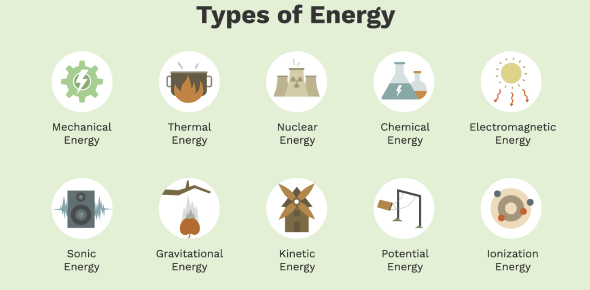# Types Of Energy Quiz! Trivia Facts

10 Questions | Attempts: 6757SettingsThis is a Types of Energy quiz. Energy is the ability to do work. There exist different types of energy sources and types, including thermal energy, radiant energy, chemical energy, nuclear energy, electrical energy, motion energy, sound energy, elastic energy, and gravitational energy. In this quiz, you will be expected to figure out which types of energy are mentioned in each question. Do give it a try and see how well you will do!

• 1.
What type of energy does the SUN give?
• A.

Mechanical

• B.

Electrical

• C.

Solar

• D.

Sound

• E.

None of the above

• 2.
What kind of energy do stereos have?
• A.

Nuclear

• B.

Mechanical

• C.

Thermal

• D.

Sound

• E.

All of the above

• 3.
• A.

Chemical

• B.

Electrical

• C.

Solar

• D.

Sound

• E.

None of the above

• 4.
What energy do plants have?
• A.

Nuclear

• B.

Chemical

• C.

Thermal

• D.

Mechanical

• E.

None of the above

• 5.
What energy do you have when you eat?
• A.

Chemical

• B.

Sound

• C.

Kinetic

• D.

Potential

• E.

None of the above

• 6.
What type of energy do missiles have?
• A.

Sound

• B.

Nuclear

• C.

Chemical

• D.

Thermal

• E.

None of the above

• 7.
What energy does fire have?
• A.

Chemical

• B.

Nuclear

• C.

Thermal

• D.

Kinetic

• E.

None of the above

• 8.
Using the pedals on your bike is what type of energy?
• A.

Chemical

• B.

Mechanical

• C.

Nuclear

• D.

Raidiant

• E.

None of the above

• 9.
Light bulbs show off what type of energy?
• A.

Raidiant

• B.

Chemical

• C.

Electrical

• D.

Sound

• E.

None of the above

• 10.
During the day the sun gives off what?
• A.

Chemical

• B.

Raidiant

• C.

Thermal

• D.

Sound

• E.

None of the above

## Related TopicsBack to top
×

Wait!
Here's an interesting quiz for you.